This book is archived and will be removed July 6, 2022. Please use the updated version.

Condensed Matter Physics

# 65 Molecular Spectra

### Learning Objectives

By the end of this section, you will be able to:

• Use the concepts of vibrational and rotational energy to describe energy transitions in a diatomic molecule
• Explain key features of a vibrational-rotational energy spectrum of a diatomic molecule
• Estimate allowed energies of a rotating molecule
• Determine the equilibrium separation distance between atoms in a diatomic molecule from the vibrational-rotational absorption spectrum

Molecular energy levels are more complicated than atomic energy levels because molecules can also vibrate and rotate. The energies associated with such motions lie in different ranges and can therefore be studied separately. Electronic transitions are of order 1 eV, vibrational transitions are of order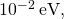and rotational transitions are of order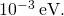For complex molecules, these energy changes are difficult to characterize, so we begin with the simple case of a diatomic molecule.

According to classical mechanics, the energy of rotation of a diatomic molecule is given by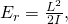where I is the moment of inertia and L is the angular momentum. According to quantum mechanics, the rotational angular momentum is quantized: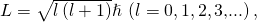where l is the orbital angular quantum number. The allowed rotational energy level of a diatomic molecule is therefore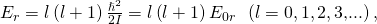where the characteristic rotational energy of a molecule is defined as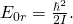For a diatomic molecule, the moment of inertia with reduced mass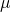is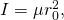where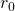is the total distance between the atoms. The energy difference between rotational levels is therefore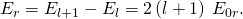A detailed study of transitions between rotational energy levels brought about by the absorption or emission of radiation (a so-called electric dipole transition) requires that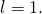This rule, known as a selection rule, limits the possible transitions from one quantum state to another. (Figure) is the selection rule for rotational energy transitions. It applies only to diatomic molecules that have an electric dipole moment. For this reason, symmetric molecules such as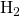and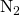do not experience rotational energy transitions due to the absorption or emission of electromagnetic radiation.

The Rotational Energy of HCl Determine the lowest three rotational energy levels of a hydrogen chloride (HCl) molecule.

Strategy Hydrogen chloride (HCl) is a diatomic molecule with an equilibrium separation distance of 0.127 nm. Rotational energy levels depend only on the momentum of inertia I and the orbital angular momentum quantum number l (in this case,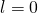, 1, and 2). The momentum of inertia depends, in turn, on the equilibrium separation distance (which is given) and the reduced mass, which depends on the masses of the H and Cl atoms.

Solution First, we compute the reduced mass. If Particle 1 is hydrogen and Particle 2 is chloride, we have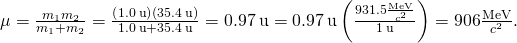The corresponding rest mass energy is therefore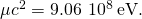This allows us to calculate the characteristic energy: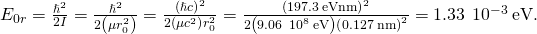(Notice how this expression is written in terms of the rest mass energy. This technique is common in modern physics calculations.) The rotational energy levels are given by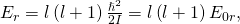where l is the orbital quantum number. The three lowest rotational energy levels of an HCl molecule are therefore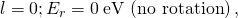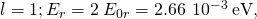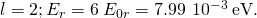Significance The rotational spectrum is associated with weak transitions (1/1000 to 1/100 of an eV). By comparison, the energy of an electron in the ground state of hydrogen is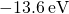.

Check Your Understanding What does the energy separation between absorption lines in a rotational spectrum of a diatomic molecule tell you?

the moment of inertia

The vibrational energy level, which is the energy level associated with the vibrational energy of a molecule, is more difficult to estimate than the rotational energy level. However, we can estimate these levels by assuming that the two atoms in the diatomic molecule are connected by an ideal spring of spring constant k. The potential energy of this spring system is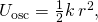Where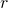is a change in the “natural length” of the molecule along a line that connects the atoms. Solving Schrödinger’s equation for this potential gives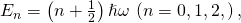Where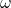is the natural angular frequency of vibration and n is the vibrational quantum number. The prediction that vibrational energy levels are evenly spaced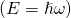turns out to be good at lower energies.

A detailed study of transitions between vibrational energy levels induced by the absorption or emission of radiation (and the specifically so-called electric dipole transition) requires that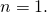(Figure) represents the selection rule for vibrational energy transitions. As mentioned before, this rule applies only to diatomic molecules that have an electric dipole moment. Symmetric molecules do not experience such transitions.

Due to the selection rules, the absorption or emission of radiation by a diatomic molecule involves a transition in vibrational and rotational states. Specifically, if the vibrational quantum number (n) changes by one unit, then the rotational quantum number (l) changes by one unit. An energy-level diagram of a possible transition is given in (Figure). The absorption spectrum for such transitions in hydrogen chloride (HCl) is shown in (Figure). The absorption peaks are due to transitions from the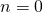to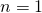vibrational states. Energy differences for the band of peaks at the left and right are, respectively,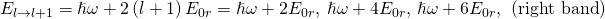and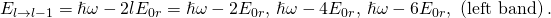The moment of inertia can then be determined from the energy spacing between individual peaks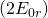or from the gap between the left and right bands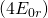. The frequency at the center of this gap is the frequency of vibration.

Three types of energy levels in a diatomic molecule: electronic, vibrational, and rotational. If the vibrational quantum number (n) changes by one unit, then the rotational quantum number (l) changes by one unit.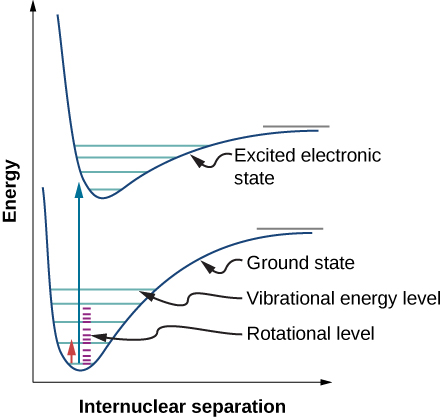Absorption spectrum of hydrogen chloride (HCl) from the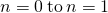vibrational levels. The discrete peaks indicate a quantization of the angular momentum of the molecule. The bands to the left indicate a decrease in angular momentum, whereas those to the right indicate an increase in angular momentum.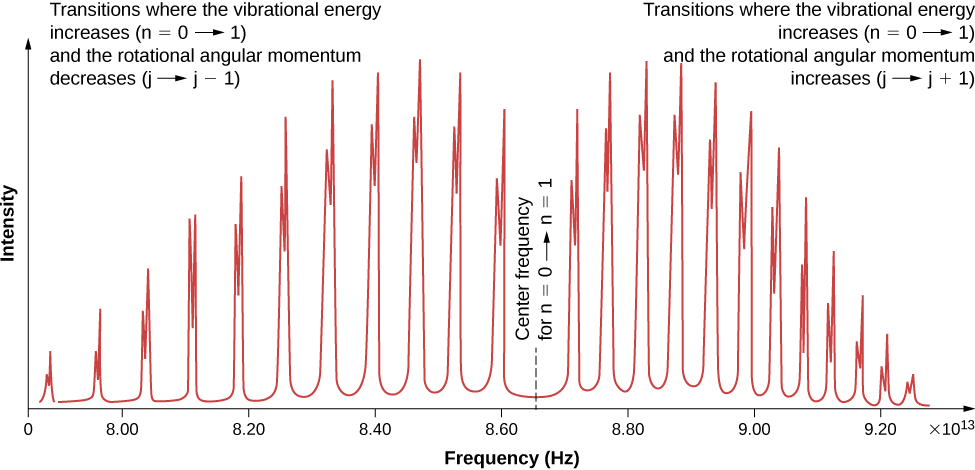### Summary

• Molecules possess vibrational and rotational energy.
• Energy differences between adjacent vibrational energy levels are larger than those between rotational energy levels.
• Separation between peaks in an absorption spectrum is inversely related to the moment of inertia.
• Transitions between vibrational and rotational energy levels follow selection rules.

### Conceptual Questions

Does the absorption spectrum of the diatomic molecule HCl depend on the isotope of chlorine contained in the molecule? Explain your reasoning.

Rank the energy spacing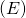of the following transitions from least to greatest: an electron energy transition in an atom (atomic energy), the rotational energy of a molecule, or the vibrational energy of a molecule?

rotational energy, vibrational energy, and atomic energy

Explain key features of a vibrational-rotation energy spectrum of the diatomic molecule.

### Problems

In a physics lab, you measure the vibrational-rotational spectrum of HCl. The estimated separation between absorption peaks is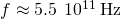. The central frequency of the band is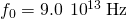. (a) What is the moment of inertia (I)? (b) What is the energy of vibration for the molecule?

For the preceding problem, find the equilibrium separation of the H and Cl atoms. Compare this with the actual value.

The measured value is 0.484 nm, and the actual value is close to 0.127 nm. The laboratory results are the same order of magnitude, but a factor 4 high.

The separation between oxygen atoms in an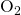molecule is about 0.121 nm. Determine the characteristic energy of rotation in eV.

The characteristic energy of themolecule is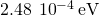. Determine the separation distance between the nitrogen atoms

0.110 nm

The characteristic energy for KCl is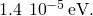(a) Determinefor the KCl molecule. (b) Find the separation distance between the K and Cl atoms.

A diatomic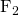molecule is in the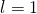state. (a) What is the energy of the molecule? (b) How much energy is radiated in a transition from ato astate?

a.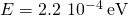; b.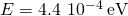In a physics lab, you measure the vibrational-rotational spectrum of potassium bromide (KBr). The estimated separation between absorption peaks is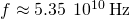. The central frequency of the band is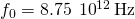. (a) What is the moment of inertia (I)? (b) What is the energy of vibration for the molecule?

### Glossary

electric dipole transition
transition between energy levels brought by the absorption or emission of radiation
rotational energy level
energy level associated with the rotational energy of a molecule
selection rule
rule that limits the possible transitions from one quantum state to another
vibrational energy level
energy level associated with the vibrational energy of a molecule